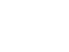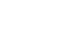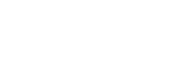## Chemistry Paper 1 Questions and Answers - Mang'u Mock 2020 Exam

CHEMISTRY
PAPER 1
TIME: 2 HOURS.

Instructions to candidates

• Answer ALL the questions in the spaces provided.
1.
1. A patient was given tablets with prescription 2 x 3 on the envelope. Clearly outline how the patient should take the tablets. (1 mark)
2. Two samples of equal volumes of water were put in 250cm beaker and heated for 10 minutes. Sample,l registered a higher temperature than sample 2.State the conditions under which flame 1 is produced in Bunsen burner.(1 mark)
2. The apparatus below was used to separate a mixture of liquid A and BState two properties of the liquids that make it possible to separate them using such apparatus. (2 marks)
3. Describe how solid samples of salts can be obtained from a mixture of lead (II) chloride, sodium chloride and ammonium chloride. (3 marks
4. An ion of element X is represented as1. Write electronic configuration of ion of x (1 mark)
2. To which group does element x belong? (1 mark)
5. The set-up below can be used to study the reaction of magnesium and steam.1. Name gas P. (1 mark)
2. How would you expect copper to behave compared to magnesium in the combustion tube? (1 mark)
3. Write the equation for the reaction between magnesium and steam (1 mark)
6. An approximately x molar solution of potassium manganite (VII) solution was standardized against precisely 0.1M iron (II) ammonium sulphate ((NH4)3 Fe(SO4)2.6H2O] solution. 25.0cm3 of the solution of the iron (II) salt were oxidized by 24.15cm3 of the manganite (VII) solution. The equation of the reaction is:

MnO4(aq)- + 5Fe(aq)2+ + 8H(aq)+  → Mn(aq)2+ + 5Fe(aq)3+ + 4H2O(l)
What is the molarity of the potassium manganite (III) solution? (3 marks)
7. During extraction of iron in the blast furnace, state the uses of the following in the furnace.
1. Molten slag. (1 mark)
2. Waste gases leaving the furnace (1 mark)
3. Limestone (1 mark)
8. The flow chart below gives some reactions starting with ethane. Study it and answer the questions that follow.1. Draw the structure of compounds
1. (1 mark)
2. (1 mark)
2.  Write the name of compound R. (1 mark)
9. Study the organic compound below:1. In which homologous series does the compound belong to? (1 mark)
2. Name and draw the structures of two compounds that can be used to prepare the above compound. (3 marks)
10.
1. State one factor that can determine the stability of an atom (1 mark)
2. Radioactive polonium - 216 decay as shown below.
216Po → 208Pb + M  + nß
90                       82
Find the value of M and n (2 marks)
3. If after 112 days, 1/16 of polonium remained, calculate the half-life of polonium (1 mark)
11. A metal oxide has a formula M203.
1. Write an equation to show how M form an ion (1 mark)
2. Write the formula of the chloride of M. (1 mark)
12. The thermodynamic equation for the formation of ammonia in the Haber process is
N2(g) + 3H2(g)2NH3(g), ΔH = -92kJ mol-1
State and explain one way in which the yield of ammonia can be increased. (2 marks)
13. A certain carbonate, JCO3, reacts with dilute hydrochloric acid according to the equation below.
JCO3 + 2HCl (aq) — → GCl(aq) + CO2(g) + H2O(l)
If 1g of the carbonate reacts completely with 20cm3 of 1M hydrochloric acid, calculate the relative atomic mass of J.
(C = 12,0= 16) (4 marks)
14.
1. What is meant by the term solubility? (1 mark)
2. The mass of a solution A is 120g. This solution has 8g of salt A dissolved in it. The solubility of this salt is 25g/100g of water at 30°C. 55g of salt A are added to the solution at 30°C. How much of salt A will remain undissolved. (2 marks)
15.
1. Using electrons in the outermost energy level, draw the dot (.) and cross (X) diagrams to represent bonding in.
1. C2H6 (C= 6, H=1) (1 mark)
2. NH4C1 (N = 7, H = 1, Cl = 17)(1 mark)
2. The formula of a complex ion is [Cu(NH3)4]2+ name the type of bond that is likely to exist between copper and ammonia in the complex (1 mark)
16.
1. State Hess's law (1 mark)
2. Study the information below and answer the questions that follow.
MgCl2(s) → Mg(g)2+ + 2Cl(g)-,           ΔH1 = -2487kJ mol-1
MgCl2(s) + (aq) → MgCl2(aq),          ΔH2 = -5142kJ Mol-1
2Cl(g)-+ (aq) → 2Cl(aq)-,                  ΔH3 = -762kJ mol-1
1. Name the enthalpies H1 and H2 (2 marks)
2. Determine the enthalpy for the reaction:
Mg(g)2+ + (aq) → Mg(g)2+     (2 marks)
17.
1. Give two reasons why carbon (IV) oxide is used as a fire extinguisher (1 mark)
2. State: the function of tartaric acid in baking powder. (2 marks)
18. When an electric current of 0.5A was passed through a molten chloride of J for 32 minutes and 10 seconds, a mass of 0.44g off J was deposited at the cathode. (IF = 96500C)
1. Calculate the quantity of electricity used. (1 mark)
2. Determine the value of x if the ion of metal J is represented as Jx+
(R.A.M of J= 14) (1 mark)
19.
1. What is meant by the term basicity of an acid? (1 mark)
2. Describe briefly how potassium sulphate can be prepared using 50cm potassium hydroxide of 1M (3 marks)
20. The diagram below represents a set-up used to prepare oxygen gas.1. Name substance Q (1 mark)
2. Complete the set up to show how oxygen gas is collected.(1mark)
3. Write the equation for the reaction that occurs. (1 mark)
21. The table below shows some solutions and their pH values
 Solution pH value P 1.5 Q 6.0 R 14.0 S 8.0
Which of the above solution
1. Is strongly basic. (1 mark)
2. Reacts with sodium carbonate more vigorously (1 mark)
3. Is ammonia solution (1 mark)
22. In an experiment, a jar containing sulphur (IV) oxide was inverted over another jar containing hydrogen sulphide gas.
1. State and explain the observation that was made. (2 marks)
2. State two conditions necessary for the reaction to take place. (2 marks)
23. Two reagents that can be used to prepare chlorine gas are potassium manganite (VII) and hydrochloric acid.
1. Write an equation for the reaction. (1 mark)
2. Give the formula of another reagent that can be used instead of potassium manganate (VII) (1 mark)
3. Using an equation illustrate how chlorine bleach coloured substances. (1 mark)
24.
1. Distinguish between ionization energy and electron affinity. (2 marks)
2. Explain why fluorine is more reactive than iodine. (2 marks)
25. 280cm3 of nitrogen gas diffuse through a porous plug in 70 seconds. How long will it take 400cm of carbon (IV) oxide gas to diffuse through the same porous plug? (C = 12, 0= 16, N = 7). (3 marks)
26. An iron spoon was to be electroplated with silver. Sketch the set-up that could be used. (2 marks)
27. Write the equation for decomposition of:
1. Sodium nitrate. (1 mark)
2. Copper (II) nitrate (1 mark)

MARKING SCHEME

1. A patient was given tablets with prescription 2 x 3 on the envelope. Clearly outline how the patient should take the tablets. (1 mark)
• Take 2 tablets after every 8 hours

2. Two samples of equal volumes of water were put in 250cm beaker and heated for 10 minutes. Sample,l registered a higher temperature than sample 2.State the conditions under which flame 1 is produced in Bunsen burner.(1 mark)
• The flame should be produced when the air hole is open

1. The apparatus below was used to separate a mixture of liquid A and BState two properties of the liquids that make it possible to separate them using such apparatus. (2 marks)
• Difference in densities
• They are immiscible

2. Describe how solid samples of salts can be obtained from a mixture of lead (II) chloride, sodium chloride and ammonium chloride. (3 marks)
• Heat to sublime ammonium chloride
• Add water to dissolve sodium chloride
• Filter the residue is lead (II) chloride .
• Evaporate the filtrate (sodium chloride solution) to obtain sodium chloride solid.

3. An ion of element X is represented as1. Write electronic configuration of ion of x (1 mark)
• 2:8

2. To which group does element x belong? (1 mark)
• Group 2 (II)

4. The set-up below can be used to study the reaction of magnesium and steam.1. Name gas P. (1 mark)
• Hydrogen

2. How would you expect copper to behave compared to magnesium in the combustion tube? (1 mark)
• Copper would not react with steam

3. Write the equation for the reaction between magnesium and steam (1 mark)
• Mg(s) + H2O(g) → MgCl(s) + H2(g)

5. An approximately x molar solution of potassium manganite (VII) solution was standardized against precisely 0.1M iron (II) ammonium sulphate ((NH4)3 Fe(SO4)2.6H2O] solution. 25.0cm3 of the solution of the iron (II) salt were oxidized by 24.15cm3 of the manganite (VII) solution. The equation of the reaction is:

MnO4(aq)- + 5Fe(aq)2+ + 8H(aq)+  → Mn(aq)2+ + 5Fe(aq)3+ + 4H2O(l)
What is the molarity of the potassium manganite (III) solution? (3 marks)
Mm = ?
Vm= 24.15cm3
MFe-0.1M
VFe = 25cm3
Mole of Fe2+ = 0.1mole = 1000cm3
? =  25cm

Mole ratio 1:5= 0.0025
moles Moles of MnO4 = 0.0025 = 0.005 moles

6. During extraction of iron in the blast furnace, state the uses of the following in the furnace.
1. Molten slag. (1 mark)
• Protect the hot iron from being re-open

2. Waste gases leaving the furnace (1 mark)
• used to preheat the air between that time base of the furnace
3. Limestone (1 mark)
• Decompose to CaO which combine with unwanted silica forming slage
7. The flow chart below gives some reactions starting with ethane. Study it and answer the questions that follow.1. Draw the structure of compounds
1. (1 mark)
2. (1 mark)2.  Write the name of compound R. (1 mark)
• Sodium ethoxide

8. Study the organic compound below:1. In which homologous series does the compound belong to? (1 mark)
• Ester

2. Name and draw the structures of two compounds that can be used to prepare the above compound. (3 marks)
1. propanoic acid
2. methanol9.
1. State one factor that can determine the stability of an atom (1 mark)
• N/P ratio
• Amount of energy released when heat collide with protons in the nucleus

2. Radioactive polonium - 216 decay as shown below.
216Po → 208Pb + M  + nß
90                       82
Find the value of M and n (2 marks)
• 216 - 208 + 4 +0
4m - 216 - 208
4m = 8/4=2
90 - 82 + 2m + -n

3. If after 112 days, 1/16 of polonium remained, calculate the half-life of polonium (1 mark)
• 1 → 1/21/41/81/16
4 half lives → 112 days
1 half life → ?
=1 x 112/4
=28 days

10. A metal oxide has a formula M203.
1. Write an equation to show how M form an ion (1 mark)
• M(g) - 3e- → M(g)3+
• M(g) → M(g)3+ + 3e-

2. Write the formula of the chloride of M. (1 mark)
• MCl3

11. The thermodynamic equation for the formation of ammonia in the Haber process is
N2(g) + 3H2(g)2NH3(g), ΔH = -92kJ mol-1
State and explain one way in which the yield of ammonia can be increased. (2 marks)
• Increase in pressure
• Withdraw of NH3(g) decrease in concentration of NH3(g) favours towards reaction
• Use of low temperature RXA of exothermic decreases in temperature forms forward reaction
• Addition of H3/N2 increase in reaction of mxns favour forward reaction
12. A certain carbonate, JCO3, reacts with dilute hydrochloric acid according to the equation below.
JCO3 + 2HCl (aq) — → GCl(aq) + CO2(g) + H2O(l)
If 1g of the carbonate reacts completely with 20cm3 of 1M hydrochloric acid, calculate the relative atomic mass of J.
(C = 12,0= 16) (4 marks)
• moles of HCl used = 1 x 20
1000 =0.02 moles
CaCO3 : HCI
1    2
Moles of CaCO3 used = 1/2 x 0.02 moles = 0.01 moles
0.01 mole = 1g
1 mole      =?
=1 x 1
0.01 = 100g

Ca + 12 + 16 + 3 = 100
Ca = 100-60
Ca=40
13.
1. What is meant by the term solubility? (1 mark)
• Solubility is the mass of a substance that can ndissolve per 100g of water

2. The mass of a solution A is 120g. This solution has 8g of salt A dissolved in it. The solubility of this salt is 25g/100g of water at 30°C. 55g of salt A are added to the solution at 30°C. How much of salt A will remain undissolved. (2 marks)
• 100g of water = 25g
112g of water = ?
112g x 25g
100g
=28g
Un dissolved salt
- (8 + 55) - 28 = 35g
14.
1. Using electrons in the outermost energy level, draw the dot (.) and cross (X) diagrams to represent bonding in.
1. C2H6 (C= 6, H=1) (1 mark)2. NH4C1 (N = 7, H = 1, Cl = 17)(1 mark)2. The formula of a complex ion is [Cu(NH3)4]2+ name the type of bond that is likely to exist between copper and ammonia in the complex (1 mark)
• Dative covalent
15.
1. State Hess's law (1 mark)
• Enthalpy change of a reaction is the same regardless of the rate followed as long as the reactants and products are the same.

2. Study the information below and answer the questions that follow.
MgCl2(s) → Mg(g)2+ + 2Cl(g)-,           ΔH1 = -2487kJ mol-1
MgCl2(s) + (aq) → MgCl2(aq),          ΔH2 = -5142kJ Mol-1
2Cl(g)-+ (aq) → 2Cl(aq)-,                  ΔH3 = -762kJ mol-1
1. Name the enthalpies H1 and H2 (2 marks)
• H1 - lattice energy
• H2 - Hydration energy of MgCl2 solution

2. Determine the enthalpy for the reaction:
Mg(g)2+ + (aq) → Mg(g)2+     (2 marks)-5142=-2489 +Hx+ -762 x 2
Hx = -5142 +2489769 = -1893kj/mol
16.
1. Give two reasons why carbon (IV) oxide is used as a fire extinguisher (1 mark)
• Denser than air
• Does not support combustion

2. State: the function of tartaric acid in baking powder. (2 marks)
• Reacts with NaHOO, to produce CO, which makes the dough to rise
• Reacts with Na C0, formed when NaHCO, is heated hence neutralize Na Co, in the dough

17. When an electric current of 0.5A was passed through a molten chloride of J for 32 minutes and 10 seconds, a mass of 0.44g off J was deposited at the cathode. (IF = 96500C)
1. Calculate the quantity of electricity used. (1 mark)
• Q = it = 0.5 x 1930 sec = 965c
2. Determine the value of x if the ion of metal J is represented as Jx+
(R.A.M of J= 14) (1 mark)
• Jx + xC. J(s) 445
965c = 0.44g
?= 44g
=96500C
X x 96500 = 965500
96500     96500
X = 1 Therefore charge +1
18.
1. What is meant by the term basicity of an acid? (1 mark)
• It is the number of replaceable hydrogen atoms in an acid

2. Describe briefly how potassium sulphate can be prepared using 50cm potassium hydroxide of 1M (3 marks)
• Mix/react 50cm3 of 0.5M H2SO4 or 25cm3 of 1M H2SO4 to obtain a neutral solution of K2SO4
• Hear to evaporate some water
• Cool slowly to crystallize the solution

19. The diagram below represents a set-up used to prepare oxygen gas.1. Name substance Q (1 mark)
• Na2O2

2. Complete the set up to show how oxygen gas is collected.(1mark)3. Write the equation for the reaction that occurs. (1 mark)
• 2Na2O2 + 2H2O(l) → 4NaOH(aq) + O2
20. The table below shows some solutions and their pH values
 Solution pH value P 1.5 Q 6.0 R 14.0 S 8.0
Which of the above solution
1. Is strongly basic. (1 mark)
• R/14.0

2. Reacts with sodium carbonate more vigorously (1 mark)
• R/1.5

3. Is ammonia solution (1 mark)
• S/8.0

21. In an experiment, a jar containing sulphur (IV) oxide was inverted over another jar containing hydrogen sulphide gas.
1. State and explain the observation that was made. (2 marks)
• Yellow solid is formed
• SO2(g) is reduced by H2S to S

2. State two conditions necessary for the reaction to take place. (2 marks)
• Jars should be moist
• The jar with the denser has should be placed on top of the jar with the gas.

22. Two reagents that can be used to prepare chlorine gas are potassium manganite (VII) and hydrochloric acid.
1. Write an equation for the reaction. (1 mark)
• 2KMnO4(s) + 16HCl(aq) → KCl + 2MnCl(g) + 8H2O(l) + 5Cl2

2. Give the formula of another reagent that can be used instead of potassium manganate (VII) (1 mark)
• MnO2

3. Using an equation illustrate how chlorine bleach coloured substances. (1 mark)
• Cl2(g) + dye + H2O(l) → 2HCl(aq) + dye - O
23.
1. Distinguish between ionization energy and electron affinity. (2 marks)
• Ionization energy is the energy required to remove an electron from a gaseous atom/ion
• Electron affinity is the energy required to add an electron to a gascous atom

2. Explain why fluorine is more reactive than iodine. (2 marks)
• Reactivity of halogens increase up the gp due to the decreased number of energy levels/ decrease in atomic radius
24. 280cm3 of nitrogen gas diffuse through a porous plug in 70 seconds. How long will it take 400cm of carbon (IV) oxide gas to diffuse through the same porous plug? (C = 12, 0= 16, N = 7). (3 marks)
• RN2 = 230
70
=4cm/sec
RCO2 = 400/t
4

400/t = √44/28
t= √44/28 x 100 = 125.36 sec.

25. An iron spoon was to be electroplated with silver. Sketch the set-up that could be used. (2 marks)26. Write the equation for decomposition of:
1. Sodium nitrate. (1 mark)
• 2NaNO3(s) → 2NaNO2(s) + O2(g)

2. Copper (II) nitrate (1 mark)
• Cu(NO3)2(s) → 2CuO(s) + 4NO2(g) +O2(g)

• ✔ To read offline at any time.
• ✔ To Print at your convenience
• ✔ Share Easily with Friends / Students